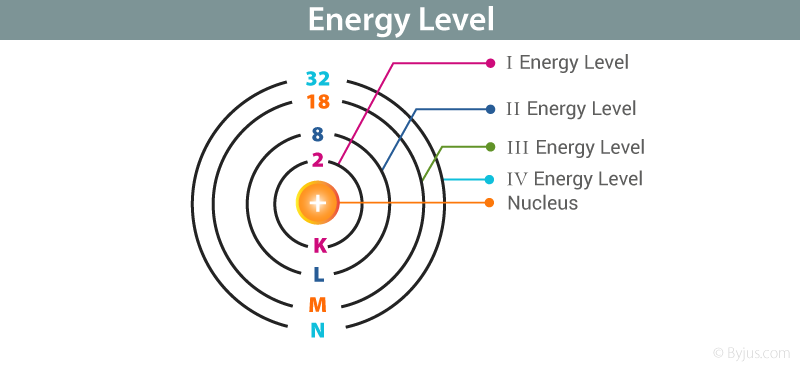Checkout JEE MAINS 2022 Question Paper Analysis : Checkout JEE MAINS 2022 Question Paper Analysis :

# Energy Level - Principle Energy Level

## Bohr’s Atomic Model:

• Thomson’s and Rutherford’s atomic models failed to answer any questions related to the energy of an atom and its stability. In the year 1913, Niels Bohr proposed an atomic model, describing an atom as a small, positively charged nucleus surrounded by electrons that travel in circular orbits around the positively charged nucleus, similar to the planets around the sun in our solar system, with attraction provided by the electrostatic forces.
• This model is popularly known as the Bohr model of an atom. Bohr proposed an atomic model of a hydrogen atom.
• Bohr’s model gave a proper explanation for the stability of electrons revolving in orbits. He named these orbits as energy shells. His postulates explaining the energy level are discussed below.### Bohr’s Explanation for Energy Level:

• Different orbits in which electrons revolve are known as stationary states or energy levels. These stationary states/ energy level for an electron are numbered as n = 1, 2, 3……….. These integers are also known as the principal quantum numbers.
• Energy of the stationary state in which an electron is placed is given by:
$$\begin{array}{l}{E}_{n} = {-R}_{h} \frac{1}{{n}^{2}}\end{array}$$

Where, RH is called Rydberg constant whose value is 2.18×10–18 J.

• The energy of an electron when it is far away from the influence of the nucleus is taken as zero. Principal quantum number of an electron existing in such a stationary state is taken as, n = ∞. Such kind of hydrogen atom is called an ionized hydrogen atom. A negative sign is placed in the above equation as, due to the transition of an electron from one orbit to another, the stationary state energy is emitted and thus, energy is lowered.
• When an electron is placed in the lowest stationary state/ energy level possible, it is said to be in the ground state. Electron in this energy level revolves in the orbit having the smallest possible radii. The energy of this state is –13.6 eV.

## Frequently Asked Questions – FAQs

### What is an atom?

An electric charge is a property of matter

An atom is the smallest possible unit of the ordinary that forms chemical elements. All solid, gas, liquid and plasma are made of ionised or neutral atoms. Atoms are very small, usually around hundred picometers across.

### What are the main components of an atom?

Atoms are predominantly made of electrons, neutrons, and protons. These particles may or may not exist depending on the nature of the atoms.

### What is an electron?

The electron is one of the main subatomic particles in an atom whose electric charge is negative one elementary charge. These particles revolve around the nucleus.

### What is a proton?

A proton is one of the main subatomic particles whose charge is positive one elementary charge. These particles are located in the nucleus of the atom.

### What is the main conclusion of Bohr’s atomic model?

Bohr developed an atomic model of a hydrogen atom. Bohr’s model provided a proper clarification for the stability of electrons revolving in fixed orbits. He termed these orbits as energy shells. Different orbits in which electrons revolve are called energy levels or stationary states. These stationary energy/state levels for an electron are denoted as n= 1, 2, 3…..

These numbers are also called the principal quantum numbers.

Test your knowledge on Energy level